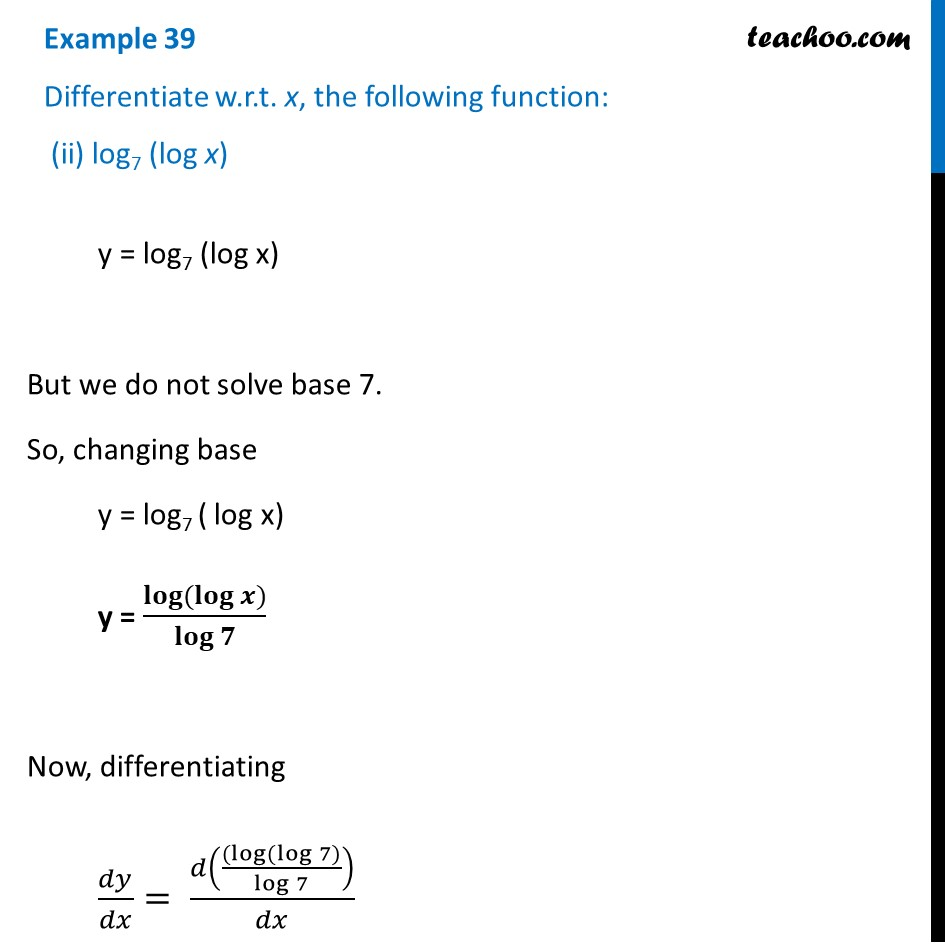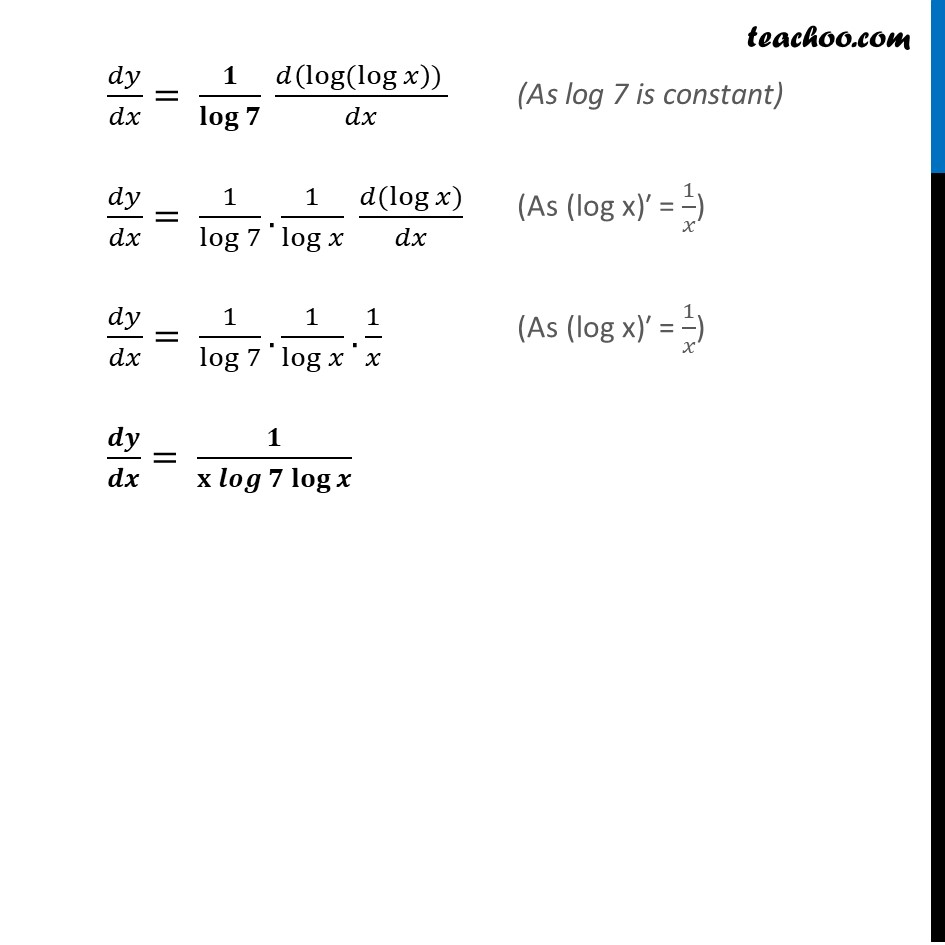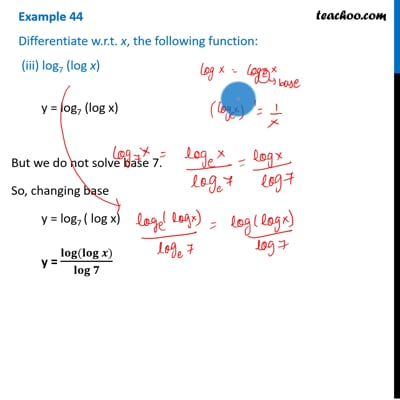Examples

Chapter 5 Class 12 Continuity and Differentiability
Serial order wiseThis video is only available for Teachoo black users

Learn in your speed, with individual attention - Teachoo Maths 1-on-1 Class

### Transcript

Example 39 Differentiate w.r.t. x, the following function: (ii) log7 (log x) y = log7 (log x) But we do not solve base 7. So, changing base y = log7 ( log x) y = 𝐥𝐨𝐠⁡〖(𝐥𝐨𝐠⁡〖𝒙)〗 〗/𝐥𝐨𝐠⁡𝟕 Now, differentiating 𝑑𝑦/𝑑𝑥= 𝑑(((log⁡〖(log⁡〖7)〗 〗)/(log 7))/𝑑𝑥 𝑑𝑦/𝑑𝑥= 𝟏/𝐥𝐨𝐠⁡𝟕 (𝑑(log⁡〖(log⁡𝑥 〗 )) )/𝑑𝑥 𝑑𝑦/𝑑𝑥= 1/log⁡7 . 1/log⁡𝑥 (𝑑(log⁡𝑥))/𝑑𝑥 𝑑𝑦/𝑑𝑥= 1/log⁡7 . 1/log⁡𝑥 . 1/𝑥 𝒅𝒚/𝒅𝒙= 𝟏/〖𝐱 𝒍𝒐𝒈〗⁡〖𝟕 𝐥𝐨𝐠⁡𝒙 〗 (As log 7 is constant) ("As (log x)′ =" 1/𝑥) ("As (log x)′ =" 1/𝑥)